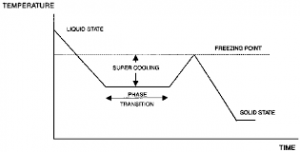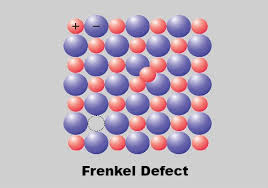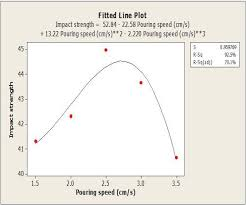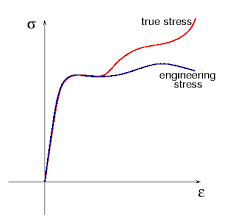## How to Calculate and Solve for Relative Freezing Time | Foundry TechnologyThe image above represents relative freezing time.

To compute for relative freezing time, four essential parameters are needed and these parameters are Constant (L), Constant (C), Volume of Riser/Volume of Casting (y) and Relative Contraction of Freezing (B).

The formula for calculating relative freezing time:

x = L / y – B + C

Where:

x = Relative Freezing Time
y = Volume of Riser / Volume of Casting
B = Relative Contraction on Freezing
C = Constant
L = Constant

Let’s solve an example;
Find the relative freezing time when the volume of riser/volume of casting is 26, the relative contraction on freezing is 21, the constant is 8 and the constant is 11.

This implies that;

y = Volume of Riser / Volume of Casting = 26
B = Relative Contraction on Freezing = 21
C = Constant = 11
L = Constant = 8

x = L / y – B + C
x = 8 / 26 – 21 + 11
x = 8 / 5 + 11
x = 1.6 + 11
x = 12.6

Therefore, the relative freezing time is 12.6 s.

Calculating the Constant (L) when the Relative Freezing Time, Constant (C), the Volume of riser/Volume of Casting and the Relative Contraction on Freezing is Given.

L = x (y – B) – C

Where;

L = Constant
x = Relative Freezing Time
y = Volume of riser / Volume of Casting
B = Relative Contraction on Freezing
C = Constant

Let’s solve an example;
Find the Constant when the relative freezing time is 24, the volume of riser / volume of casting is 14, the relative contraction on freezing is 8 and the constant is 10.

This implies that;

x = Relative Freezing Time = 24
y = Volume of riser / Volume of Casting = 14
B = Relative Contraction on Freezing = 8
C = Constant = 10

L = x (y -B) – C
L = 24 (14 – 8) – 10
L = 24 (6) – 10
L = 144 – 10
L = 134

Therefore, the constant is 134.

## How to Calculate and Solve for Frenkel Defect | CeramicsThe image above represents frenkel defect.

To compute for frenkel defect, four essential parameters are needed and these parameters are N, activation energy (Qfr), Boltzmann’s Constant (K) and temperature (T).

The formula for calculating the frenkel defect:

Nfr = N exp (-Qfr / 2KT)

Where:

Nfr = Frenkel Defect
Qfr = Activation Energy
K = Boltzmann’s Constant
T = Temperature

Let’s solve an example;
Find the frenkel defect when the activation energy is 34, N is 22, Temperature is 12 and the boltzmann’s constant is 1.38064852e-23.

This implies that;

Qfr = Activation Energy
K = Boltzmann’s Constant
T = Temperature

Nfr = N exp (-Qfr / 2KT)
Nfr = (22)exp(-(34) / 2(1.38064852e-23)(14))
Nfr = (22)exp((-34) / (3.865815856e-22))
Nfr = (22)exp(-8.795038684325786e+22)
Nfr = (22)(0)
Nfr = 0

Therefore, the frenkel defect is 0.

Calculating the Activation Energy when the Frenkel Defect, the Boltzmann’s Constant and the Temperature is Given.

Qfr = – (In (Nfr / N) x 2KT)

Where;

Qfr = Activation Energy
Nfr = Frenkel Defect
K = Boltzmann’s Constant
T = Temperature

Let’s solve an example;
Given that the frenkel defect is 20, the boltzmann’s constant is 5, the temperature is 2 and N is 10. Find the activation energy?

This implies that;

Nfr = Frenkel Defect = 20
K = Boltzmann’s Constant = 5
T = Temperature = 2
N = 10

Qfr = – (In (Nfr / N) x 2KT)
Qfr = – (In (20 / 10) x 2 x 5 x 2)
Qfr = – (In 2 x 20)
Qfr = – (In 40)
Qfr = – 3.688

Therefore, the activation energy is – 3.688.

## How to Calculate and Solve for Pouring Speed | Design of Gating SystemThe image above represents pouring speed.

To compute for poring speed, three essential parameters are needed and these parameters are Co-efficient for friction (μ), Acceleration due to Gravity (g) and Rated head static (Hs).

The formula for calculating pouring speed:

v = μ√(2gHs)

Where:

v = Pouring Speed
μ = Co-efficient for Friction
g = Acceleration due to Gravity
Hs = Rated Static Head

Let’s solve an example;
Find the pouring speed when the co-efficient for friction is 14, acceleration due to gravity is 9 and rated static head is 16.

This implies that;

μ = Co-efficient for Friction = 14
g = Acceleration due to Gravity = 9
Hs = Rated Static Head = 16

v = μ√(2gHs)
v = 14 x √(2 x 9 x 16)
v = 14 x √(288)
v = 14 x 16.97
v = 237.58

Therefore, the pouring speed is 237.58 m/s.

Calculating the Co-efficient for Friction when the Pouring Speed, the Acceleration due to Gravity and the Rated Static Head is Given.

μ = v / √(2gHs)

Where;

μ = Co-efficient for Friction
v = Pouring Speed
g = Acceleration due to Gravity
Hs = Rated Static Head

Let’s solve an example;
Find the co-efficient for friction when the pouring speed is 24, the acceleration due to gravity is 9 and the rated static head is 11.

This implies that;

v = Pouring Speed = 24
g = Acceleration due to Gravity = 9
Hs = Rated Static Head = 11

μ = v / √(2gHs)
μ = 24 / √(2 x 9 x 11)
μ = 24 / √198
μ = 24 / 14.07
μ = 1.70

Therefore, the co-efficient for friction is 1.70.

## How to Calculate and Solve for True Stress | The Calculator EncyclopediaThe image above represents the true stress.

To compute for the true stress, two essential parameters are needed and these parameters are force (F) and instantaneous area (Ai).

The formula for calculating true stress:

σT = F / Ai

Where;
T = True Stress
F = Force
Ai = Instantaneous Area

Let’s solve an example;
Find the true stress when the instantaneous area is 60 with a force of 25.

This implies that;
F = Force = 25
Ai = Instantaneous Area = 60

σT = F / Ai
σT = 25 / 60
σT = 0.416

Therefore, the true stress is 0.416 Pa.

Calculating the Force when True Stress and Instantaneous Area is Given.

F = Ai x σT

Where;
F = Force
σT = True Stress
Ai = Instantaneous Area

Let’s solve an example;
Find the force when the instantaneous area is 30 with a true stress of 15.

This implies that;
σT = True Stress = 15
Ai = Instantaneous Area = 30

F = Ai x σT
F = 30 x 15
F = 450

Therefore, the force is 450.